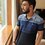# A Curious Series!

I was dwindling with the Basel series and thinking of ways to prove it. I did this: $\displaystyle \sum_{n=0}^{\infty} \dfrac{1}{n^{2}+1} = \int_{0}^{1} \sum_{n=0} ^{\infty} x^{n^{2}}dx$ So, the series is: $\displaystyle \sum_{n=0}^{\infty} x^{n^{2}}$ for $$|x| <1$$ Wolfram Alpha gives the result in terms of Jacobi Theta Functions, which I currently fail to comprehend, So, what are your views on it??

And, more generally for the series: $\displaystyle \sum_{n=0}^{\infty} x^{n^{\alpha}}$ where, $\alpha$ is a positive integer or maybe a real, and $|x|<1$Note by Kunal Gupta
5 years, 9 months ago

This discussion board is a place to discuss our Daily Challenges and the math and science related to those challenges. Explanations are more than just a solution — they should explain the steps and thinking strategies that you used to obtain the solution. Comments should further the discussion of math and science.

When posting on Brilliant:

• Use the emojis to react to an explanation, whether you're congratulating a job well done , or just really confused .
• Ask specific questions about the challenge or the steps in somebody's explanation. Well-posed questions can add a lot to the discussion, but posting "I don't understand!" doesn't help anyone.
• Try to contribute something new to the discussion, whether it is an extension, generalization or other idea related to the challenge.

MarkdownAppears as
*italics* or _italics_ italics
**bold** or __bold__ bold
- bulleted- list
• bulleted
• list
1. numbered2. list
1. numbered
2. list
Note: you must add a full line of space before and after lists for them to show up correctly
paragraph 1paragraph 2

paragraph 1

paragraph 2

[example link](https://brilliant.org)example link
> This is a quote
This is a quote
    # I indented these lines
# 4 spaces, and now they show
# up as a code block.

print "hello world"
# I indented these lines
# 4 spaces, and now they show
# up as a code block.

print "hello world"
MathAppears as
Remember to wrap math in $$ ... $$ or $ ... $ to ensure proper formatting.
2 \times 3 $2 \times 3$
2^{34} $2^{34}$
a_{i-1} $a_{i-1}$
\frac{2}{3} $\frac{2}{3}$
\sqrt{2} $\sqrt{2}$
\sum_{i=1}^3 $\sum_{i=1}^3$
\sin \theta $\sin \theta$
\boxed{123} $\boxed{123}$

Sort by:

- 5 years, 9 months ago

Your question is not clear. What do we need to do? It is Jacobi functions, yeah. You want to know about what Jacobi Theta is or other methods to solve the problem?

BTW, you can use Laplace transform too.

- 5 years, 9 months ago

@Kartik Sharma Sorry, for mingling the prob like this , but what I want in particular is the summation of that particular series and that if it can be put up in a closed form other than that of jacobi functions.My point is not emphasized on Jacobi functions, but the "series"

- 5 years, 9 months ago

I don't mean to ruin your fun but if there's a simpler way to evaluate $\displaystyle \sum_n q^{n^2}$ without using Jacobi Theta functions, mathematicians would have known about it already.

- 5 years, 9 months ago

This is experience.

Ramanujan "guessed" it, why can't we? (This is based on the fact that many people believe that Ramanujan guessed almost every one of his results ans didn't provide results... He should not be regarded as a true mathematician(sarcasm of course)).

- 5 years, 9 months ago

The only thing that I can think of is Rogers-Ramanujan Identities but I doubt that's even relevant to the question at hand.

Yeah, Pierre de Fermat is not a mathematician either (sarcasm).

- 5 years, 9 months ago

Okay, now the following is random but I just wanted to know more about Skewes' number and more about the shift of inequality sign of $\pi(x)$ and $\text{Li}(x)$, can you help?

- 5 years, 9 months ago

I assumed you've watched this video already. I only know as much as you.

Whether Riemann hypothesis is true or not, the inequality $\pi(n) < \text{li}(n)$ will fail for a number lower than $e^{e^{e^{79}}}$ or for a number larger than $10^{10^{10^{10^3}}}$. Since I'm not verse in Logarithmic Integral, I doubt I can push further than this.

- 5 years, 9 months ago

@Kartik Sharma I got: $\displaystyle \int_{0}^{\infty} \dfrac{\sin(mx)dx}{1-e^{-x}} = \dfrac{1}{2m} + \dfrac{\pi}{2}\coth(\pi m)$

- 5 years, 9 months ago

Yeah. BTW, yeah, I am thinking about the series only for a moment. I too have not yet got any chance to enjoy Theta functions but have heard a lot about them. I would wish to learn about them at the end of my course with Wilfred Kaplan's book(here course means self study of Kaplan). But yeah, I wish to think of these without any theta.

- 5 years, 9 months ago

I don't know why you want to convert it into an integral (which is wrong by the way). You can simply solve it via digamma functions or residues to get the answer of $\frac12(\pi \coth(\pi) + 1)$.

- 5 years, 9 months ago

@Pi Han Goh It's not about the sum provided in the beginning, it's about the series given at the end

- 5 years, 9 months ago